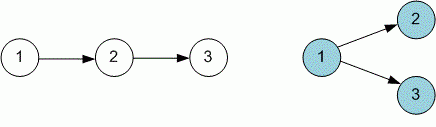Competitions

The unique Topological Sort

The directed unweighted graph is given. Find out if it has a unique topological ordering of its vertices.

Input

The first line contains the number of vertices n (1n2 * 105) and the number of edges m (1m105) in a graph. Each of the next m lines describes the edge of the graph - two numbers, the initial and final vertex.

Output

Print "YES" if the vertices of the graph can be uniquely lexicographically sorted and "NO" otherwise. If it is impossible to sort graph topologically, print -1.Time limit 1 second
Memory limit 128 MiB
Input example #1
3 2
1 2
2 3
Output example #1
YES
Input example #2
3 2
1 2
1 3
Output example #2
NO
Author Michael Medvediev